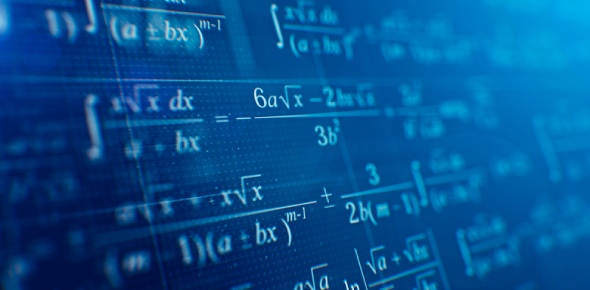# Mathematics

3 Questions | Total Attempts: 42SettingsTry to solve the following test. . Its sooo easy:p

Related Topics
• 1.
2x+3y=2 4x-3y=4 FIND Y
• A.

-1

• B.

1

• C.

0

• D.

2

• E.

Not from the above

• 2.
A(2,5) B(3,5) find vector AB coordinate
• A.

AB(1,2)

• B.

AB(2,1)

• C.

AB(0,2)

• D.

AB(1,0)

• E.

AB(0,0)

• 3.
(x squared + 4)=??
• A.

-2

• B.

+2

• C.

+1

• D.

0

• E.

It has more than one solution